Ex 3.7

Chapter 3 Class 6 Playing with Numbers
Serial order wise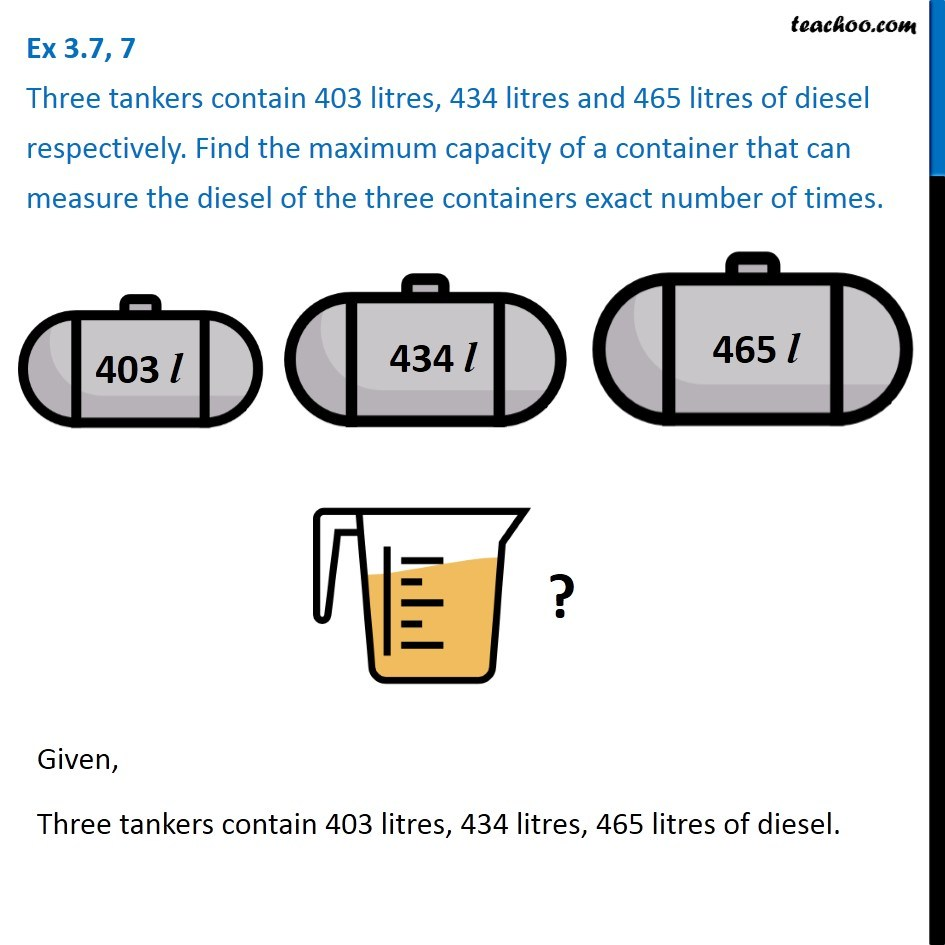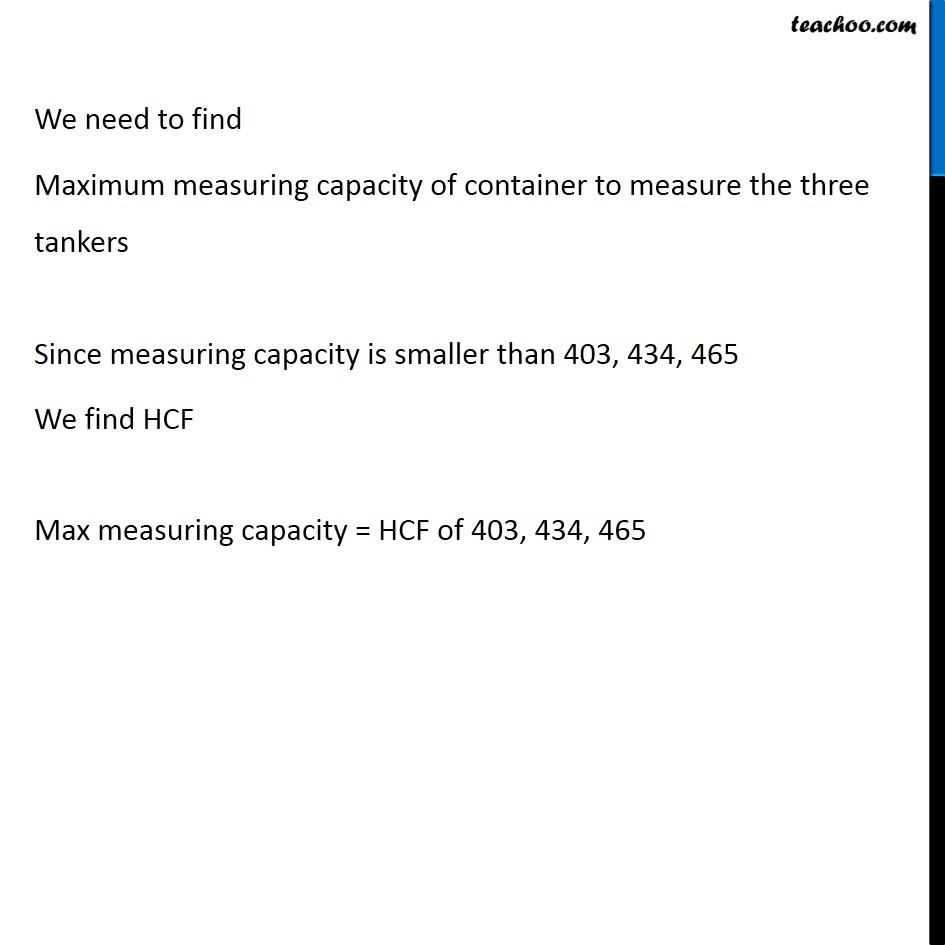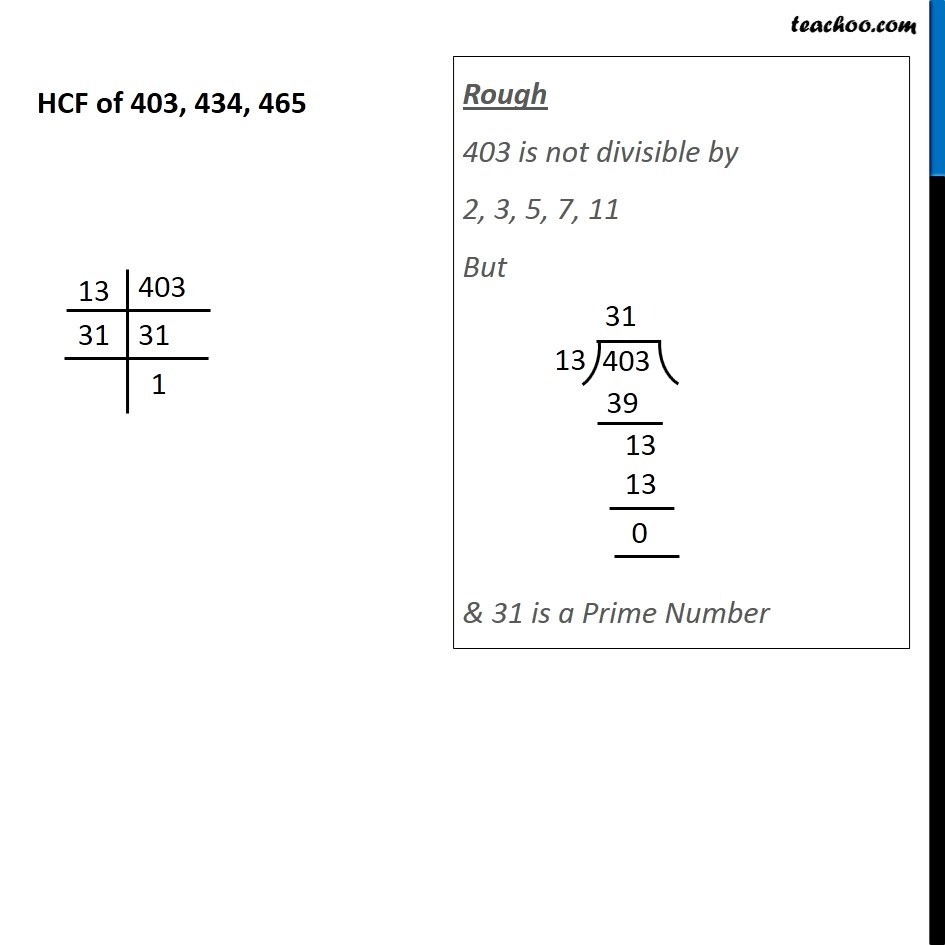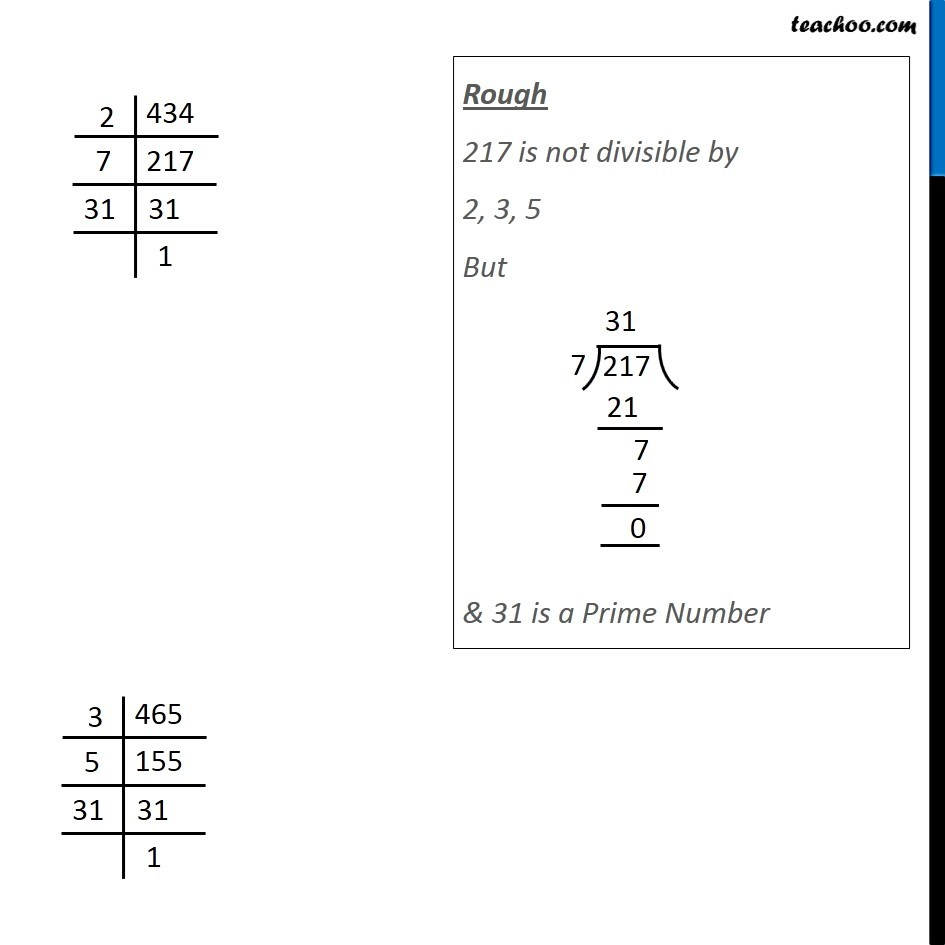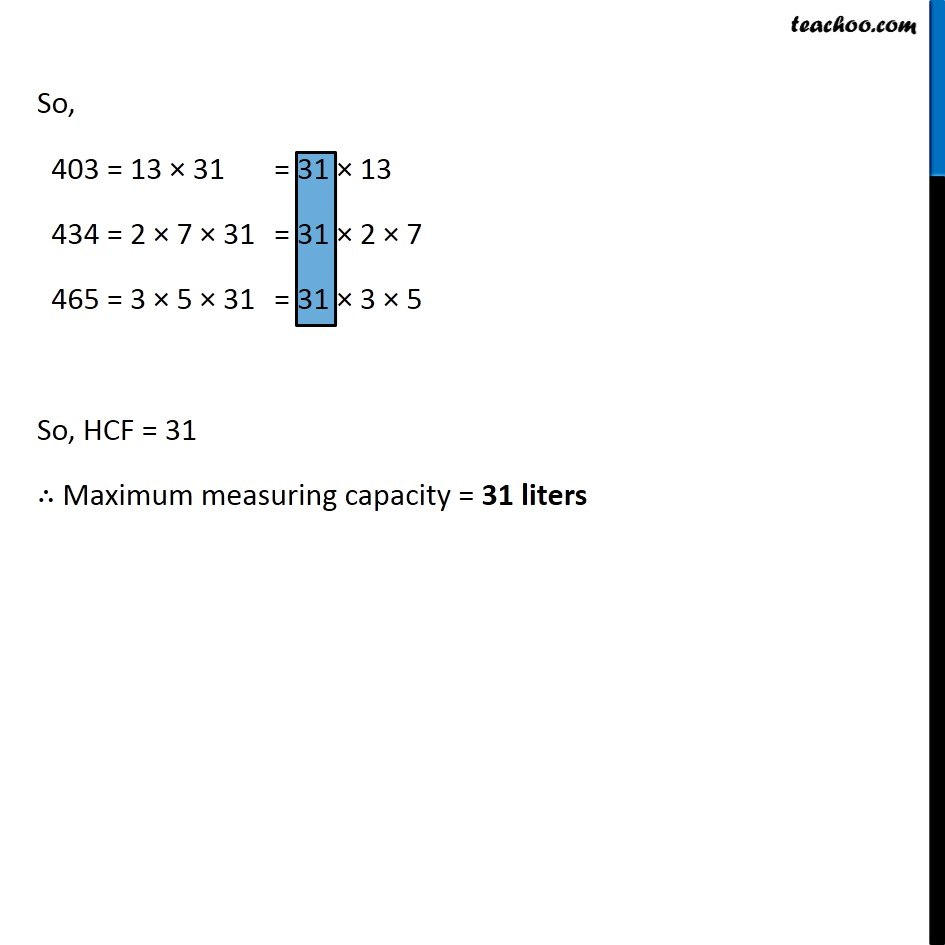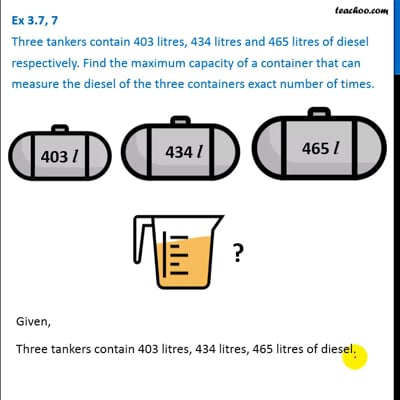This video is only available for Teachoo black users

### Transcript

Ex 3.7, 7 Three tankers contain 403 litres, 434 litres and 465 litres of diesel respectively. Find the maximum capacity of a container that can measure the diesel of the three containers exact number of times. Given, Three tankers contain 403 litres, 434 litres, 465 litres of diesel. We need to find Maximum measuring capacity of container to measure the three tankers Since measuring capacity is smaller than 403, 434, 465 We find HCF Max measuring capacity = HCF of 403, 434, 465 Rough 403 is not divisible by 2 2, 3, 5, 7, 11 But & 31 is a Prime Number Rough 217 is not divisible by 2, 3, 5 But & 31 is a Prime NumberRough 217 is not divisible by 2, 3, 5 But & 31 is a Prime Number So, 403 = 13 × 31 434 = 2 × 7 × 31 465 = 3 × 5 × 31 So, HCF = 31 ∴ Maximum measuring capacity = 31 liters